# A mixture of table salt and ice is used to chill the contents of hand-operated ice-cream makers. What is the melting point of a mixture of 2.00 lb of NaCl and 12.00 lb of ice if exactly half of the ice melts? Assume that all the NaCl dissolves in the melted ice and that the van't Hoff factor for the resulting solution is 1.44.

Question
1 views

A mixture of table salt and ice is used to chill the contents of hand-operated ice-cream makers. What is the melting point of a mixture of 2.00 lb of NaCl and 12.00 lb of ice if exactly half of the ice melts? Assume that all the NaCl dissolves in the melted ice and that the van't Hoff factor for the resulting solution is 1.44.

check_circle

Step 1

Given, the van't hoff factor for the resulting solution = 1.44

The amount of NaCl = 2.0 lb

The amount of ice (H2O) =12.00 lb

The amount of NaCl in terms of kilogram is represented as -

1 lb = 0.4535 Kg

2.0 lb = 2.0 x 0.4535 Kg

= 0.907 Kg

It is given that exactly half of the ice melts. Therefore, the amount of ice (H2O) is expressed as -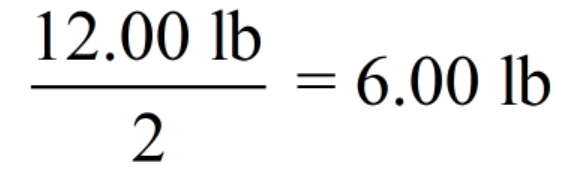The amount of ice (H2O) in terms of kilogram is represented as -

1 lb = 0.4535 Kg

6.0 lb = 6.0 x 0.4535 Kg

= 2.721 Kg

Step 2

The change in freezing point (ΔTf) is represented as -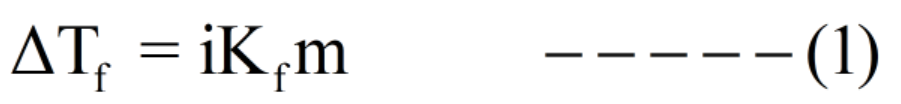Now we will calculate the molality -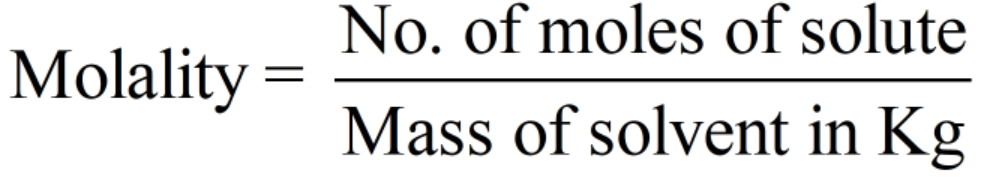The number of moles (n) of NaCl is calculated by the formula -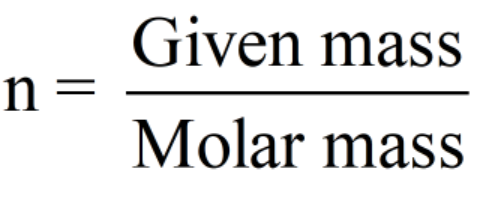Molar mass of NaCl = 58.44 g/mol

Substituting the given mass and molar mass,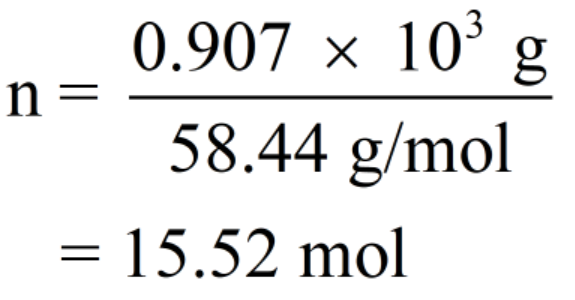Step 3

The given mass of solute ice (H2O) = 2.721 Kg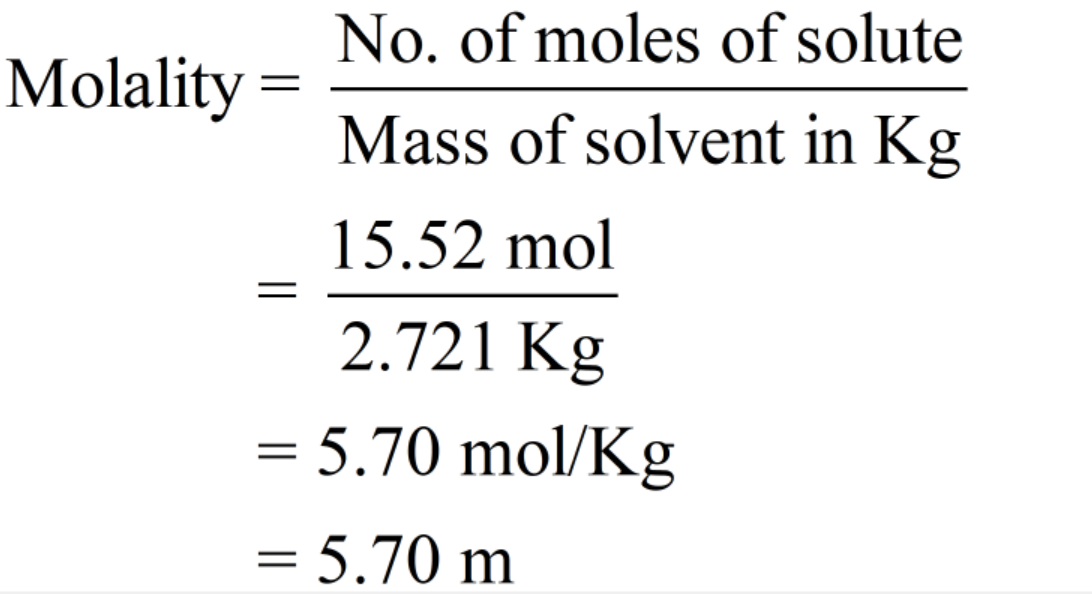The value of freezing point depression constant = 1.86°C/m

...

### Want to see the full answer?

See Solution

#### Want to see this answer and more?

Solutions are written by subject experts who are available 24/7. Questions are typically answered within 1 hour.*

See Solution
*Response times may vary by subject and question.
Tagged in

### Chemistry